# Low Resolution Proton NMR Spectra

$$\newcommand{\vecs}{\overset { \rightharpoonup} {\mathbf{#1}} }$$ $$\newcommand{\vecd}{\overset{-\!-\!\rightharpoonup}{\vphantom{a}\smash {#1}}}$$$$\newcommand{\id}{\mathrm{id}}$$ $$\newcommand{\Span}{\mathrm{span}}$$ $$\newcommand{\kernel}{\mathrm{null}\,}$$ $$\newcommand{\range}{\mathrm{range}\,}$$ $$\newcommand{\RealPart}{\mathrm{Re}}$$ $$\newcommand{\ImaginaryPart}{\mathrm{Im}}$$ $$\newcommand{\Argument}{\mathrm{Arg}}$$ $$\newcommand{\norm}{\| #1 \|}$$ $$\newcommand{\inner}{\langle #1, #2 \rangle}$$ $$\newcommand{\Span}{\mathrm{span}}$$ $$\newcommand{\id}{\mathrm{id}}$$ $$\newcommand{\Span}{\mathrm{span}}$$ $$\newcommand{\kernel}{\mathrm{null}\,}$$ $$\newcommand{\range}{\mathrm{range}\,}$$ $$\newcommand{\RealPart}{\mathrm{Re}}$$ $$\newcommand{\ImaginaryPart}{\mathrm{Im}}$$ $$\newcommand{\Argument}{\mathrm{Arg}}$$ $$\newcommand{\norm}{\| #1 \|}$$ $$\newcommand{\inner}{\langle #1, #2 \rangle}$$ $$\newcommand{\Span}{\mathrm{span}}$$

This page describes how you interpret simple low resolution nuclear magnetic resonance (NMR) spectra. It assumes that you have already read the background page on NMR so that you understand what an NMR spectrum looks like and the use of the term "chemical shift".

## Difference between high and low resolution spectra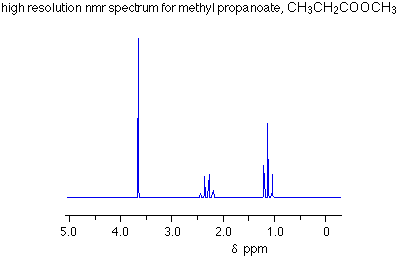A low resolution spectrum looks much simpler because it cannot distinguish between the individual peaks in the various groups of peaks.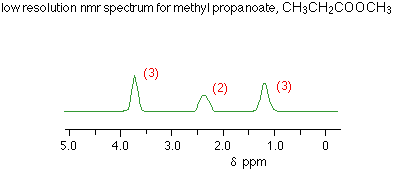The numbers against the peaks represent the relative areas under each peak. That information is extremely important in interpreting the spectra.

## Interpreting a low resolution spectrum

### Using the total number of peaks

Each peak represents a different environment for hydrogen atoms in the molecule. In the methyl propanoate spectrum above, there are three peaks because there are three different environments for the hydrogens. Remember that methyl propanoate is CH3CH2COOCH3. The hydrogens in the CH2 group are obviously in a different environment from those in the CH3 groups. The two CH3 groups aren't in the same environment either. One is attached to a CH2 group, the other to an oxygen.

### Using the areas under the peaks

The ratio of the areas under the peaks tell you the ratio of the numbers of hydrogens in the various environments. In the methyl propanoate case, the areas were in the ratio of 3:2:3, which is exactly what you want for the two differently placed CH3 groups and the CH2 group.

You will probably be told the relative areas under the peaks - especially if you are only looking at low resolution spectra, but it is just possible that you might have to work them out. NMR spectrometers have a device which draws another line on the spectrum called an integrator trace (or integration trace). You can measure the relative areas from this trace.

### Using chemical shifts

The position of the peaks tells you useful things about what groups the various hydrogen atoms are in. The important shifts for the groups present in methyl propanoate are: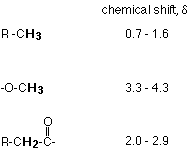Showing these groups on the low resolution spectrum gives: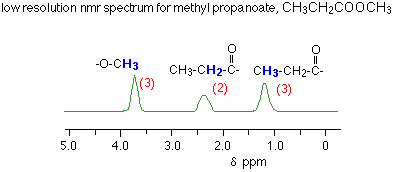## Questions

1. An organic compound was known to be one of the following. Use its low resolution NMR spectrum to decide which it is.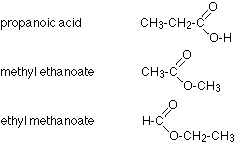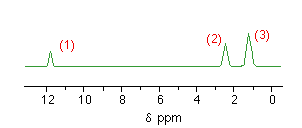Notice that there are three peaks showing three different environments for the hydrogens. That eliminates methyl ethanoate as a possibility because that would only give two peaks - due to the two differently situated CH3 group hydrogens.

Does the ratio of the areas under the peaks help? Not in this case - both the other compounds would have three peaks in the ratio of 1:2:3. Now you need to look at the chemical shifts: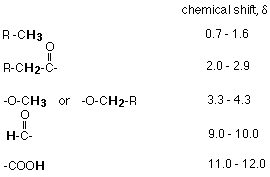Checking the positions of the various hydrogens in the two possible compounds against the chemical shift table gives you this pattern of shifts:Comparing these with the actual spectrum means that the substance was propanoic acid, CH3CH2COOH.2. How would you use low resolution NMR to distinguish between the isomers propanone and propanal?The propanone would only give one peak in its NMR spectrum because both CH3 groups are in an identical environment - both are attached to -COCH3. The propanal would give three peaks with the areas underneath in the ratio 3:2:1. You could refer to the chemical shift table above to decide where the peaks are likely to be found, but it isn't really necessary.

3. How many peaks would there be in the low resolution NMR spectrum of the following compound, and what would be the ratio of the areas under the peaks?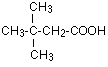All the CH3 groups are exactly equivalent so would only produce 1 peak. There would also be peaks for the hydrogens in the CH2 group and the COOH group. There would be three peaks in total with areas in the ratio 9:2:1.